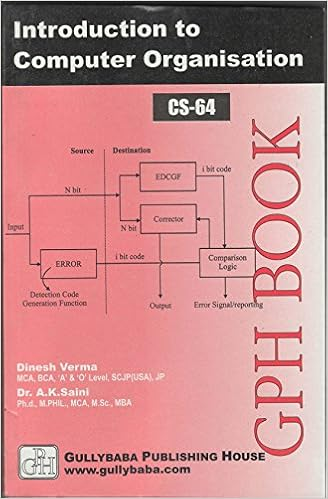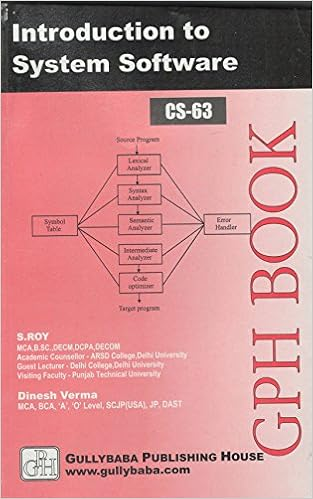IGNOU BCA MCA.comQuestion Papers of IGNOU BCA CS62

 CS62: 'C' programming and Data StructureYear: 2003 TEE: june Time 2 Full Marks 60 Note: There are 6 questions in this paper. Question 1 is compulsory. Answer any three questions from the rest. All algorithms should be written nearer to ?c? language.Q.1(b): Given two Sorted Lists L1 and L2 write algorithm to compute L1UL2. L1 and L2 are to be implemented as arrays. Q.1(c): Write a function that takes only a pointer to the root of a binery tree T and computes the number of nodes in T.: Q.2(a): Draw the internal memory representation of the following Binary Tree using Sequential Representation. Assume that nodes appear in the following physical sequence:A, B, C, D, E, G, F, H: Q.2(b): Consider the tree in the following figure:Give the Postfix Prefix and Infix expressions corresponding to the above tree.: home || Next >>